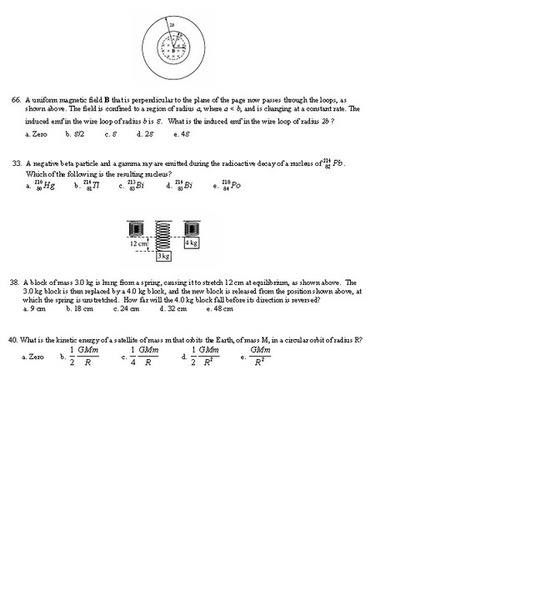# Physics Practice test questions

## Homework Statement## The Attempt at a Solution

in progress..

66. $$\varepsilon = Blv \qquad \varepsilon=\frac{-\Delta\phi_{m}}{\Delta t} \qquad \phi_{m}=BAcos\Theta=\;\pi r^{2}\qquad 4\pi b^{2}$$ That's why I chose (e) for 66.

33. I have no idea on this one. I just picked the one that had an atomic number and mass number lower than the beginning nucleus.

38. I tried using mgh=1/2kx^2 . I found (k) first by using F=ma on the mass in equilibrium but ended up with a huge number. Is my method correct?

Last edited:

Is this statement correct?:
The classical model of an ideal gas:
• The molecules are in random motion.
• The volume of the molecules is negligible com¬pared with the volume occupied by the gas.
• The molecules obey Newton's laws of motion.
• The collisions between molecules are elastic
• The only appreciable forces on the molecules are those that occur during collisions.

Astronuc
Staff Emeritus
Is this statement correct?:
The classical model of an ideal gas:
• The molecules are in random motion.
• The volume of the molecules is negligible compared with the volume occupied by the gas.
• The molecules obey Newton's laws of motion.
• The collisions between molecules are elastic
• The only appreciable forces on the molecules are those that occur during collisions.

What do you think? Why would each of the five statements be true or not?

What would be the impact if molecules were not in random motion?

What would be the effect of inelastic collision between molecules?

Astronuc
Staff Emeritus
66. $$\varepsilon = Blv \qquad \varepsilon=\frac{-\Delta\phi_{m}}{\Delta t} \qquad \phi_{m}=BAcos\Theta=\;\pi r^{2}\qquad 4\pi b^{2}$$ That's why I chose (e) for 66.

33. I have no idea on this one. I just picked the one that had an atomic number and mass number lower than the beginning nucleus.

38. I tried using mgh=1/2kx^2 . I found (k) first by using F=ma on the mass in equilibrium but ended up with a huge number. Is my method correct?

$$\varepsilon=\frac{-\Delta\phi_{m}}{\Delta t}$$ is correct, but which variable ($B,\,A,\,\theta$) is changing?

$$\phi_{m}=BAcos\Theta=\;\pi r^{2}$$ does this equality makes sense?

The magnetic field is confined to a < b, and we now that the inductance in loop of radius b is $\epsilon$, so what of the larger loop?

33. In beta decay, the mass number (A, number of nucleons) remains the same, but the charge of the nucleus (Z) must increase by 1 to (Z+1), because a neutron decays to a proton, electron and anti-neutrino.

38. One can determine the k from the 3 kg mass, which is at (static) equilibrium. F = kx. The 4 kg mass is released, so in addition to its weight, there is a brief acceleration until the spring force exceeds the weight and restores the system. One does apply conservation of energy in this situation.

40. One can use the balance of gravitational force with centripetal force to determine the orbital velocity from which one can determine the kinetic energy.

http://library.thinkquest.org/03oct/02144/basics/orbittal.htm

For a low orbiting satellite, the altitude is much less than the radius of the earth.

http://www.glenbrook.k12.il.us/gbssci/phys/Class/circles/u6l4c.html [Broken]

and also - Lesson 4: Planetary and Satellite Motion
Energy Relationships for Satellites
http://www.glenbrook.k12.il.us/gbssci/phys/Class/circles/u6l4e.html [Broken]

Last edited by a moderator:
What do you think? Why would each of the five statements be true or not?

What would be the impact if molecules were not in random motion?

What would be the effect of inelastic collision between molecules?

I think that they are true because if you heat up an object then the energy gets distributed throughout the entire object and no just a few molecules. If the molecules collided inelastically they would stick together and soon there would be only the random motion of 1 big molecule?